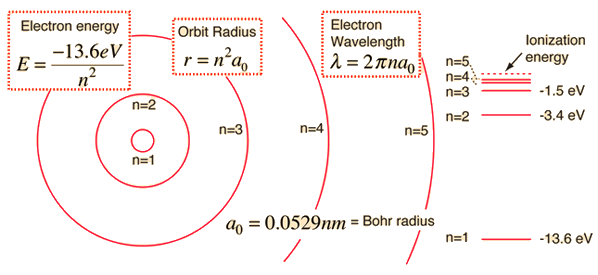# What is ΔE for the transition of an electron from n = 7 to n = 4 in a Bohr hydrogen atom? What is the frequency, v, of the spectral line produced?

Jun 12, 2016

$\left(a\right)$

$9.16 \times {10}^{- 20} \text{ ""J}$

$\left(b\right)$

$1.38 \times {10}^{14} {\text{ ""s}}^{- 1}$

#### Explanation:

$\left(a\right)$

The Bohr Model for the energy of an electron in a hydrogen atom gives us:

$E = \frac{- 13.6}{{n}^{2}} \text{ ""eV}$

Where $n$ is the principle quantum number.For the transition $7 \rightarrow 4$ the difference in energy is given by:

$\Delta E = - \frac{13.6}{7} ^ 2 - \left[- \frac{13.6}{4} ^ 2\right] \text{ ""eV}$

$\Delta E = \frac{13.6}{16} - \frac{13.6}{49} \text{ ""eV}$

$\Delta E = 0.85 - 0.277 = 0.572 \text{ ""eV}$

To convert this to Joules we multiply by the electronic charge:

$\Delta E = 0.572 \times 1.6 \times {10}^{- 19} = 9.16 \times {10}^{- 20} \text{ ""J}$

$\left(b\right)$

To find the frequency of the emitted photon we use the Planck expression:

$\Delta E = h \nu$

$\therefore \nu = \frac{\Delta E}{h} = \frac{9.16 \times {10}^{- 20}}{6.626 \times {10}^{- 34}} {\text{ ""s}}^{- 1}$

$\nu = 1.38 \times {10}^{14} {\text{ ""s}}^{- 1}$